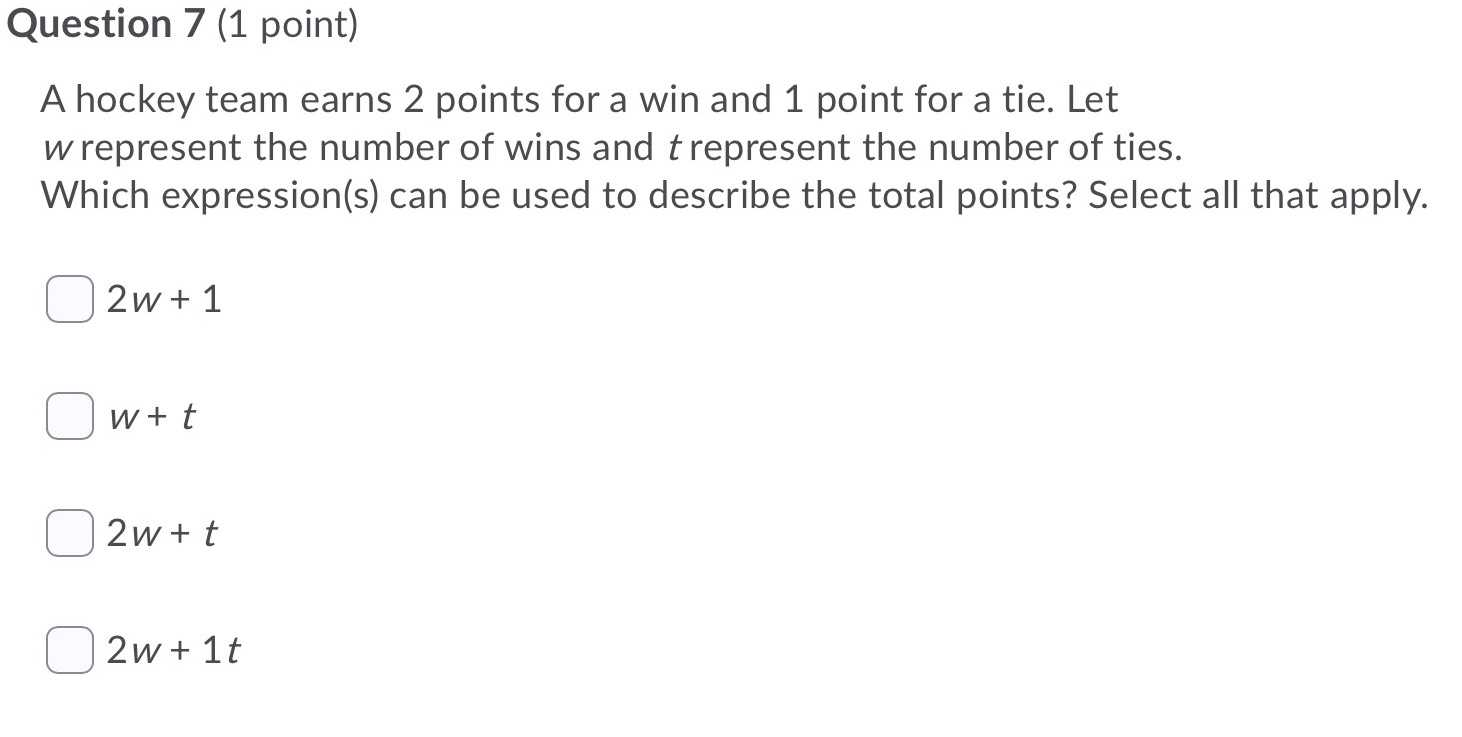### ¿Todavía tienes preguntas de matemáticas?

Pregunte a nuestros tutores expertos
Algebra
PreguntaA hockey team earns $$2$$ points for a win and $$1$$ point for a tie. Let $$w$$ represent the number of wins and $$t$$ represent the number of ties. Which expression(s) can be used to describe the total points? Select all that apply.

$$\square 2 w + 1$$

$$\square w + t$$

$$\square 2 w + t$$

$$\square 2 w + 1 t$$